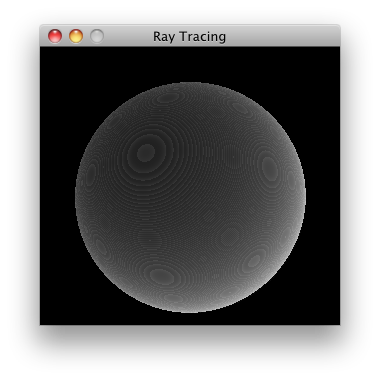## Ray-Tracing in Clojure

Following is a port of the ray-tracer from ANSI Common Lisp, by Paul Graham to Clojure. Ray-tracing is a simple rendering algorithm which yields realistic images but it is computationally expensive.

The idea is that, we trace rays of light from the eye back through the image plane into the scene, each ray is tested to see if it hits any of the objects on the scene, if the ray misses all the objects on the scene than the pixel is shaded the background color, if it hits an object then the pixel is set to the color value returned by the ray.

(defstruct v3d-struct :x :y :z)

(defn v3d [x y z]
(struct v3d-struct x y z))

(defn sq [x]
(* x x))

(defn sqrt [x]
(Math/sqrt x))

(defn magnitude [u]
(sqrt (apply + (map sq (vals u)))))

(defn normalize [u]
(let [mag (magnitude u)]
(apply v3d (map #(/ % mag) (vals u)))))

(defn subtract [u v]
(apply v3d (map #(- %1 %2) (vals u) (vals v))))

(defn distance [u v]
(magnitude (subtract u v)))

(defn minroot [a b c]
(if (zero? a)
(/ (- c) b)
(let [disc (- (sq b) (* 4 a c))]
(if (> disc 0)
(let [discroot (sqrt disc)]
(min (/ (+ (- b) discroot) (* 2 a))
(/ (- (- b) discroot) (* 2 a))))))))


Before we begin we need to define some vector utilities, all of the above functions should be self explanatory except minroot, which solves the quadratic equation.

(defstruct sphere-struct :color :radius :center)

(defn sphere [v r c]
(struct sphere-struct c r v))

(defn sphere-normal [s pt]
(normalize (subtract (:center s) pt)))

(defn sphere-intersect [s pt ray]
(let [c (:center s)
a (+ (sq (:x ray)) (sq (:y ray)) (sq (:z ray)))
b (* 2 (+ (* (- (:x pt) (:x c)) (:x ray))
(* (- (:y pt) (:y c)) (:y ray))
(* (- (:z pt) (:z c)) (:z ray))))
c (+ (sq (- (:x pt) (:x c)))
(sq (- (:y pt) (:y c)))
(sq (- (:z pt) (:z c)))
(- (sq (:radius s))))
n (minroot a b c)]

(if n
(v3d (+ (:x pt) (* n (:x ray)))
(+ (:y pt) (* n (:y ray)))
(+ (:z pt) (* n (:z ray)))))))


Next we define functions to determine where a sphere gets hit with a ray and the surface normal of a hit.

(defn lambert [s intersection ray]
(let [normal (sphere-normal s intersection)]
(max 0 (+ (* (:x ray) (:x normal))
(* (:y ray) (:y normal))
(* (:z ray) (:z normal))))))

(defn first-hit [world pt ray]
(->> (reduce (fn[h v]
(if-let [i (sphere-intersect v pt ray)]
(conj h [i v]) h)) [] world)
(sort-by #(distance (first %) pt))
first))

(defn send-ray [world src ray]
(if-let [[loc obj] (first-hit world src ray)]
(* (lambert obj loc ray) (:color obj))
0))

(defn color-at [world eye x y]
(let [ray (normalize (subtract (v3d x y 0) eye))]
(send-ray world eye ray)))

(defn ray-trace [world eye w h]
(let [buffered-image (java.awt.image.BufferedImage.
w h java.awt.image.BufferedImage/TYPE_BYTE_GRAY)
coords (for [x (range 1 w) y (range 1 h)] [x y])
colors (pmap #(let [[x y] %]
[x y (color-at world eye x y)]) coords)]
(doseq [[x y c] colors]
(.setRGB buffered-image x y
(.getRGB (java.awt.Color.
(float c) (float c) (float c)))))
buffered-image))


We iterate each pixel on the image and calculate a color for it, to do this send-ray has to find the object it reflected from, for that it calls first-hit which iterates through all the objects in the world and finds the one the ray hit first (if any). To find the amount of light shining on the surface, we refer to Lambert's law which says that the intensity of light reflected by a point on a surface is proportional to the dot-product of the unit normal vector N at that point, and the unit vector L from the point to the light source.

(defn view [image]
(doto (javax.swing.JFrame. "Ray Tracing")
(.add (proxy [javax.swing.JPanel] []
(paintComponent [g]
(proxy-super paintComponent g)
(.drawImage g image 0 0 this))))
(.setSize (.getWidth image) (.getHeight image))
(.setResizable false)
(.setVisible true)))


Once the image is rendered all thats left to do is to paint it on a panel,

(comment

(let [eye (v3d 150 150 200)
world [(sphere (v3d 150 150 -600) 400 0.8)]
image (ray-trace world eye 300 300)]
(view image))

(let [eye (v3d 150 150 200)
world [(sphere (v3d 150 150 -600) 400 0.85)
(sphere (v3d 250 200 -600) 400 0.85)
(sphere (v3d 200 100 -600) 400 0.65)
]
image (ray-trace world eye 300 300)]
(view image))

)